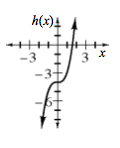### Home > CCA2 > Chapter Ch1 > Lesson 1.2.1 > Problem1-59

1-59.

Make a table and graph for $h(x)=x^3−4$ . Find the domain, range, and intercepts.

Make a table with values of $x$ between $−3$ and $3$; see if that gives you enough of a picture of the graph.
For the domain, what possible $x$-values can you substitute for $x$?
For the range, what possible $y$-values can appear in the $h(x)$ column?
One of the intercepts appears in the table and is exact. Which one?
You can see the other intercept on your graph, but it is approximate.
Use the equation to find a more precise answer by substituting $0$ for
one of the variables.

$\left. \begin{array} { c | c } { x } & { h ( x ) } \\ \hline - 3 & { - 31 } \\ { - 2 } & { - 12 } \\ { - 1 } & { - 5 } \\ { 0 } & { - 4 } \\ { 1 } & { - 3 } \\ { 2 } & { 4 } \\ { 3 } & { 23 } \end{array} \right.$Use the table in the eTool below to find the domain, range, and intercepts.
Click the link at right for the full version of the eTool: CCA2 1-59 HW eTool